# C++ Introduction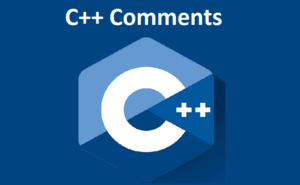C++ Comments: In this tutorial, we will find out about C++ comments, why we use them, and how to use ...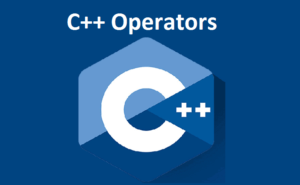#### C++ Operators

C++ Operators: In this tutorial, we will find out about the various sorts of operators in C++ with the help ...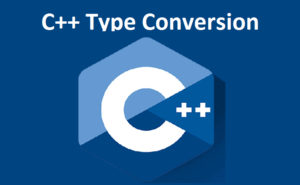#### C++ Type Conversion

C++ Type Conversion: In this tutorial, we will find out about the essentials of C++ type conversion with the help ...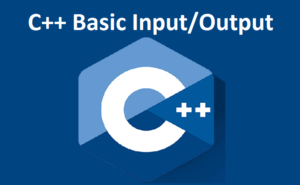#### C++ Basic Input/Output

C++ Basic Input/Output C++ Input/Output In this tutorial, we will learn to use the cin item to take input from ...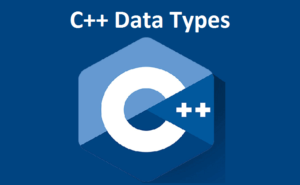#### C++ Data Types

C++ Data Types: In this tutorial, we will find out about fundamental data types, for example, int, float, char, and ...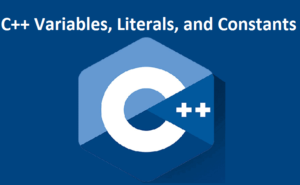#### C++ Variables, Literals, and Constants

C++ Variables, Literals and Constants: In this tutorial, we will find out about variables, literals, and constants in C++ with ...